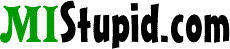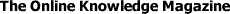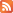# Order of Operations

When performing mathematical equations, there are rules to determine the order in which different mathematical operations are to take place. Unlike reading where we simply read left to right, the entire equation must be examined and processed according to these "order of operations."

1. Calculations must be done from left to right.

2. Calculations in brackets (parenthesis) are done first. When there are more than one set of brackets, do the inner brackets first.

3. Exponents (i.e. 32) must be done next.

4. Multiply and divide in the order the operations occur.

5. Add and subtract in the order the operations occur.

Examples:

Using only left to right...
3 + 4 x 2 = 14
Incorrect

Following correct order of operations...
3 + 4 x 2 = 11
Correct

## Mnemonic:

Please Excuse My Dear Aunt Sally
(Parenthesis, Exponents, Multiply, Divide, Add, Subtract)

When in doubt, you can't use too many parenthesis.

Equation Calculator
=
Enter an equation. Example: ((2*(6-1))/2)*4

Operators
 Home | Advertising | Donate | Contribute | Link to Us | Privacy | Contact | About |© 2001-2023 MIStupid.com